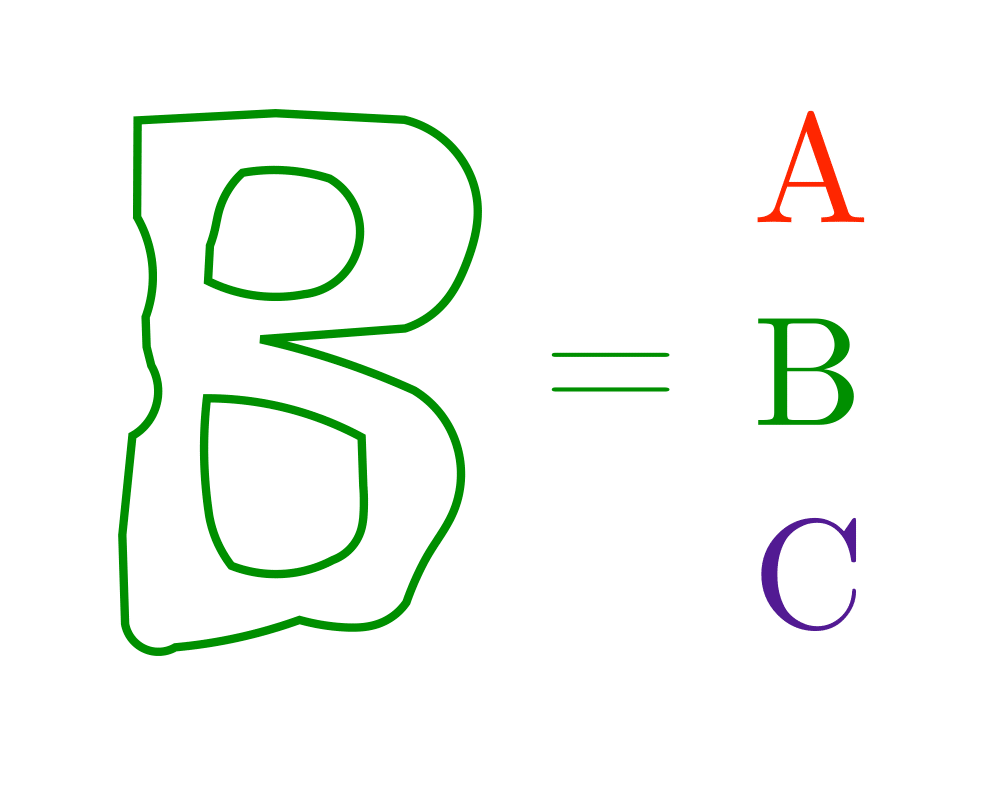# Shape SimilarityInputOutput

Input Description: Two polygonal shapes, $$P_1$$ and $$P_2$$.
Problem: How similar are $$P_1$$ and $$P_2$$?

Excerpt from The Algorithm Design Manual: Shape similarity is a problem that underlies much of pattern recognition. Consider a system for optical character recognition (OCR). We have a known library of shape models representing letters and the unknown shapes we obtain by scanning a page. We seek to identify an unknown shape by matching it to the most similar shape model.

The problem of shape similarity is inherently ill-defined, because what similar'' means is application dependent. Thus no single algorithmic approach can solve all shape matching problems. Whichever method you select, expect to spend a large chunk of time tweaking it so as to achieve maximum performance. Don't kid yourself -- this is a difficult problem.

Go To Main Page